## CircumcenterThe center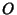of a Triangle's Circumcircle. It can be found as the intersection of the Perpendicular Bisectors. If the Triangle is Acute, the circumcenter is in the interior of the Triangle. In a Right Triangle, the circumcenter is the Midpoint of the Hypotenuse.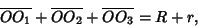(1)

whereare the Midpoints of sides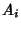,is the Circumradius, andis the Inradius (Johnson 1929, p. 190). The Trilinear Coordinates of the circumcenter are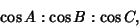(2)

and the exact trilinears are therefore(3)

The Areal Coordinates are(4)

The distance between the Incenter and circumcenter is. Given an interior point, the distances to the Vertices are equal Iff this point is the circumcenter. It lies on the Brocard Axis.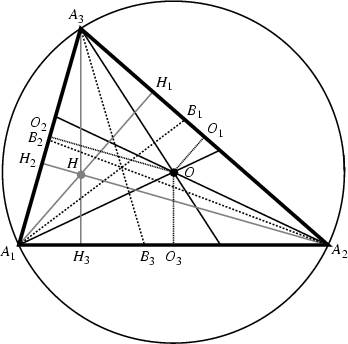The circumcenterand Orthocenterare Isogonal Conjugates.The Orthocenterof the Pedal Triangle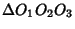formed by the Circumcenterconcurs with the circumcenteritself, as illustrated above. The circumcenter also lies on the Euler Line.

See also Brocard Diameter, Carnot's Theorem, Centroid (Triangle), Circle, Euler Line, Incenter, Orthocenter

References

Carr, G. S. Formulas and Theorems in Pure Mathematics, 2nd ed. New York: Chelsea, p. 623, 1970.

Dixon, R. Mathographics. New York: Dover, p. 55, 1991.

Eppstein, D. Circumcenters of Triangles.'' http://www.ics.uci.edu/~eppstein/junkyard/circumcenter.html.

Johnson, R. A. Modern Geometry: An Elementary Treatise on the Geometry of the Triangle and the Circle. Boston, MA: Houghton Mifflin, 1929.

Kimberling, C. Central Points and Central Lines in the Plane of a Triangle.'' Math. Mag. 67, 163-187, 1994.

Kimberling, C. Circumcenter.'' http://cedar.evansville.edu/~ck6/tcenters/class/ccenter.html.